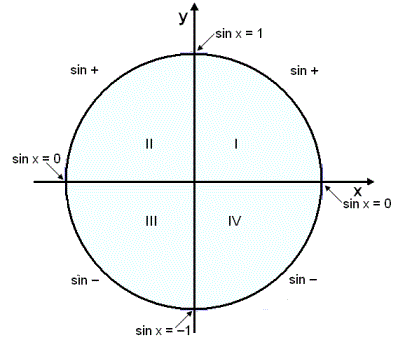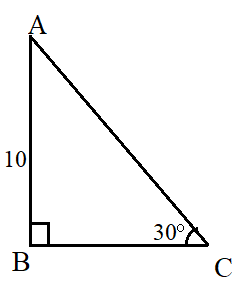## Sine Calculator

#### Sine Calculator

Result:

 sin(X): 0

### What is sine?

Sine is a trigonometric function. In a right-angled triangle, the sine of an angle x is defined as the ratio of the side opposite to the angle, to the hypotenuse.

$$sin\, x = {opposite \over hypotenuse}$$

Consider the right-angled triangle below.In this triangle, the right angle is at B. We wish to find the sine of x. The side opposite the angle x is AB, and the hypotenuse is AC. Therefore,

$$sin\, x\, =\, {opposite \over hypotenuse} \,=\,{AB\over AC}$$

### Properties of the sine function

Given below are some properties of the sine function.

Angle – Denoted by a variable x or θ, this is the parameter for which the sine value is calculated.

Domain – The values of the angle x for which we can compute sin(x). This value goes from -infinitive to +infinitive.

Range – The values between which sin(x) of any angle x lies. This value is -1≤ sin(x) ≤1.

Period – Horizontal length of one complete cycle of the sine function. The period for sin(x) is 2π radians or 360⁰.

Given below is a graph of the sine function.The sine function is periodic in nature, with a period of 2π radians (or 360⁰). This means that, the graph repeats itself every 2π radians. In the above graph, the x axis denotes the angle, and the y-axis denotes sine of that angle.

From the graph, it can be seen that sin(x) goes from 0 to +1, and then it falls to -1. From there, it goes upwards again. It attains the value +1 at π/2 radians and -1 at 3π/2 radians.

### Values of sin(x)

Given below are some commonly used values of sin(x).

Angle x in degrees Angle x in radians Sin(x)
0 0 0
30 $$π\over6$$ $$1\over2$$
45 $$π\over4$$ $$1\over√2$$
60 $$π\over3$$ $$√3\over2$$
90 $$π\over2$$ 1
120 $$2π\over3$$ $$√3\over2$$
135 $$3π\over4$$ $$1\over√2$$
150 $$5π\over6$$ $$1\over2$$
180 π 0
270 $$3π\over2$$ -1
360 0

The value of sin(x) can be positive or negative, depending on where the angle x lies in the four quadrants.
For example, sin(150⁰) is positive, whereas sin(270⁰) is negative. This has been illustrated below.

Quadrant x in degrees x in radians Sign of the sine function Range of sin values
1 0⁰ to 90⁰ $$0\, to\, {π\over2}$$ + 0 < sin(x) < 1
2 90⁰ to 180⁰ $${π\over2}\, to\, π$$ + 0 < sin(x) < 1
3 180⁰ to 270⁰ $$π\, to\, {3π\over2}$$ - -1 < sin(x) < 0
4 270⁰ to 360⁰ $${3π\over2}\, to\, 2π$$ - -1 < sin(x) < 0

If the angle x does not lie between 0⁰ to 360⁰ (or 0 to 2π radians), it can be expressed as a multiple, sum or difference of one of the above angles, and then its sine value can be determined.### Sine formula

To calculate sin(x) from a right-angled triangle, the formula used is

$$sin\, x = {opposite \over hypotenuse}$$

A different formula is used when we wish to calculate the sine of an angle in a calculator. This can be done using the Taylor series approximation for the sine function, when the angle x is in radians. The formula is;

$$sin\, x = x - {x^3\over3!} + {x^5\over5!} - {x^7\over7!} + {x^9\over9!} - ...$$

The more terms taken, the more accurate is the approximation.

### Areas of application

Trigonometric functions like the sine function can be used to model several real-life scenarios, such as electric currents, tides and radio waves. Real-life problems involving harmonic motion can be solved using the sine function, for example, the propagation of sound waves in a medium. The sin formula is also frequently used to solve trigonometric problems or prove trigonometric identities.

In this section, we consider some example problems involving the use of the sine formula.

Question: A ladder rests against a wall, making an angle of 30⁰ with the horizontal. If the height of the wall is 10 feet, what is the length of the ladder?

Answer:This problem can be visualized as below.The ladder is denoted by AC, whose length we need to find out. The wall is denoted by AB.

• Height of the wall = 10 feet
• Using the sine formula, we can compute the length of the ladder.

• sin 30 = opposite / hypotenuse = AB / AC = 10 / AC
• sin 30 = 10/AC <==> 1/2 = 10/AC
• Hence, AC = 20 feet.

The ladder is 20 feet long.

In the next example, we use the Taylor series formula for sine to find the value of an angle.

Question: Find the value of sin 25⁰ ?

Answer:For using the Taylor series formula, the angle x must be converted to radians. To convert 25⁰ to radians, we multiply by π and divide by 180.

Angle x in radians = 25π/180=0.43633

$$sin\, x = x - {x^3\over3!} + {x^5\over5!} - {x^7\over7!} + {x^9\over9!} - ...$$

$$sin\, 0.43633 = 0.43633 - {0.43633^3\over3!} + {0.43633^5\over5!} - {0.43633^7\over7!} + {0.43633 ^9\over9!} - ...$$

sin⁡ 0.43633 = 0.43633 - 0.013845 + 0.000132 - 0 + 0 - …

sin⁡0.43633 = 0.422617

Hence, sin 25⁰≈0.4226

Now, consider the case of an angle which does not lie between 0⁰ and 360⁰.

Question: Find the value of sin 540⁰ ?

Answer: The angle 540⁰ does not lie between 0⁰ and 360⁰. We try to reduce the value of this angle by removing all the full rotations of 360⁰.

Now, 540⁰ = 360⁰ + 180⁰.

Removing one full rotation shows us that sin 540⁰ is equivalent to sin 180⁰.

From the sine graph, as well as the table of listed values, we can see that sin 180⁰ = 0.

Hence, sin 540⁰ = 0.